# The Dynamics of Bolide Entry and Fragmentation in the Earth's Atmosphere

## Introduction

This report summarizes the dynamics of a bolide entering the Earth's atmosphere prior to a possible impact with the surface. This includes the loss of the object's initial kinetic energy to ablation and deceleration due to atmospheric drag as well as the possible fragmentation of the object in the atmosphere. We present a numerical procedure to compute various quantities of interest, such as the object's speed or energy dissipation as functions of time or altitude above the surface, given a set of initial parameters. Finally this analysis is applied to the Tunguska event of 1908 and the Lugo bolide of 1993.

The term bolide has no technical definition, but generally refers to extraterrestrial bodies up to 10 km in size of various possible compositions - rocky or metallic asteroid, comet, and others - entering the atmosphere with speeds in the thousands of km/sec according to US Geological Survey. We examine a range of possible bolide compositions and kinematic initial parameters (speed, angle of trajectory, etc.).

## Dynamics of a bolide prior to fragmentation

The change in an object's kinetic energy, E = m v2/2, is written as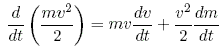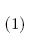Where the first term in the case of a bolide describes atmospheric deceleration. If v is the speed of the object, then in time dt the front of the object sweeps a volume proportional to Av*dt and the object's momentum decreases by an amount proportional to the momentum of air molecules contained in this volume, &rhoaAv2. In fact,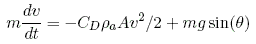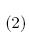Here h is the object's altitude above the ground, CD is the drag coefficient, &rhoa is the atmospheric density at altitude h, A is the cross-sectional area of the object (viewed in direction of motion). The second term corresponds to the acceleration due to gravity g = G Mearth/(Rearth + h)2 at altitude h along the direction of motion, where &theta is the instantaneous angle of the trajectory as measured from the horizon. For the Earth's atmosphere we have &rhoa = &rho0exp(-h/H), where &rho0 = 1.293 kg/m3 is the atmospheric density at sea level (h = 0) and H = 8 km is an atmospheric scale height .

The second term in equation 1 corresponds to mass loss due to ablation as the surface of the bolide is heated by radiation emitted by the shock front of gas [3,4]. As the object descends through the atmosphere, a portion of the kinetic energy of the molecules in the atmosphere vaporizes mass dm in time dt. Since in time dt the object sweeps a volume proportional to Av*dt, we expect dm/dt to be proportional to &rhoaAv3/2, the kinetic energy of the mass of air impinging upon the bolide. In fact ,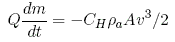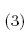where Q is the material-dependent heat of ablation, [Q] = J/kg, and CH is a dimensionless heat transfer coefficient, measured experimentally. Chyba et. al  observe that for large objects such as bolides there is an upper limit on dm/dt because such objects ablate primarily due to thermal radiation emitted by the shock wave of gas immediately preceding the object. The temperature of this gas is roughly 25,000 K, only weakly dependent on object size, speed, and composition, so that the maximum ablation rate is Qdm/dt = A&sigmaT4, where &sigma is the Stefan-Boltzmann constant. Thus we rewrite equation 3 as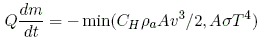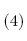The trajectory angle of the bolide varies as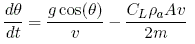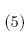where CL is the dimensionless lift coefficient (~0.001 based on crater field investigations). Finally we may write the vertical and horizontal components of the bolide's velocity, respectively, as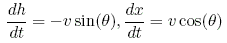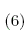We have assumed so far that the earth is flat, but for a non-infinite radius of curvature Rearth Passey and Melosh provide corrections to equations 5 and 6 that are of order h/Rearth.

## Dynamics of Bolide After Fragmentation

Bolide fragmentation is not fully understood and different authors have used different sets of assumptions and approximations in numerically modeling this process [1-3]. We may at least observe that fragmentation begins when the pressure on the leading face of the object due to the compressed air exceeds the strength of the object. As we noted above, the momentum transferred to the leading face of the bolide is proportional to &rhoaAv2, and in fact this face experiences a pressure ps = CD&rhoav2/2, where CD is a dimensionless drag coefficient (~0.5 for a rough sphere, Passey and Melosh). The air pressure on the bolide perpendicular to its direction of motion and on its back is much smaller than ps , so we approximate the average pressure inside the bolide by ps/2. The condition for fragmentation is then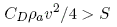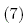where S is the yield strength of the object, [S] = N/m2, measured experimentally for various bolide compositions and is typically in the range 105-108 N/m2. When equation 7 holds, elastic failure occurs and the bolide begins to deform outwards, transversely to the direction of motion. An approximation to the force balance on the sides of the object is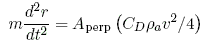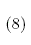where r is radius of the bolide transverse to the direction of motion and α is a dimensionless geometric constant of order unity. This is the approximation used by Chyba et. al , where α = 2π for a square cylinder (and CD = 1.7). In the case of a spherical bolide we calculate α = π2 by integrating total transverse pressure over the surface of a sphere. Since the mass of a spherical bolide is (4/3)πR3ρm, assuming that the bolide deforms due to rearrangement of its mass rather than its compression - that is, ρm is constant - we derive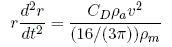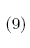where our factor of 16/3π is 2 for Chyba et. al's cylinder, but is of the same order of magnitude in this approximation. This is the approach to fragmentation we implement in the numerical simulations we describe below.

## Numerical modeling

After specifying initial speed, angle of trajectory, altitude, mass, and density - the latter two sufficient to determine cross-sectional area A - we integrate simultaneously eqs. 2, 4, 5, and 6 until the fragmentation condition, eq. 7 is true. Past this point we use eq. 9 to calculate the transverse radius r and the cross-sectional area A = πr2.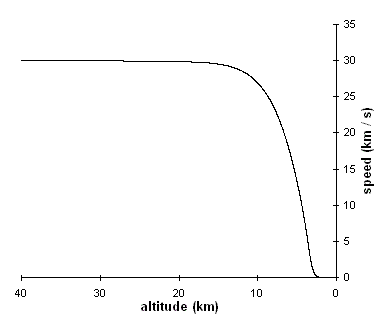Fig. 1: Speed vs. altitude for stony impactor at θ = 30°.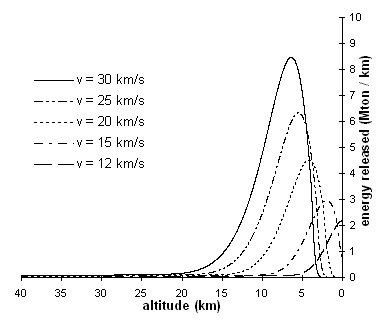Fig. 2: dEkin/dh vs. altitude for different initial v, stony impactors with θ0= 30°.

This numerical integration is implemented by discretizing time into steps of size dt and updating the other dynamic variables (mass, speed, etc.) at every time step. The simplest way to do this is to write zn+1 = zn + z'dt, where z' is an approximation of dzn/dt, most easily obtained by z'=(zn-zn-1)/dt. This method accumulates an error of order dt in the course of the simulation. We do better in accuracy, but slower in computation time, by implementing a fourth-order Runge-Kutta method to achieve an accumulated error of order dt4. Please see the C++ code for implementation details.

## Results of Computation

Figures 1-4 present the sorts of data we can collect by running the simulation. Of particular interest are the speed of the bolide and the kinetic energy released by the deceleration and ablation of the bolide. Since it is somewhat arbitrary when we begin our simulation so long as the bolide is high enough (we use 100 km for the edge of the Earth's atmosphere, in accordance with [1-3]), the relevant variable to plot against is not time, but altitude. A natural energy scale turns out to be a MTon of TNT.

Fig. 1 shows the speed of a stony bolide with initial mass of 5.6*108 kg, kinetic energy 60 MTon, trajectory angle &theta = 30°, which fragmented at 21.4 km above the ground according to the simulation. Though not apparent from the figure, the speed of the object tends to its free-fall speed, roughly 55 m/s in this case, rather than 0. Objects with initial energies on the order of tens of megatons lose most of their kinetic energy over the altitude difference of only about 10 km, as is apparent from Fig. 2.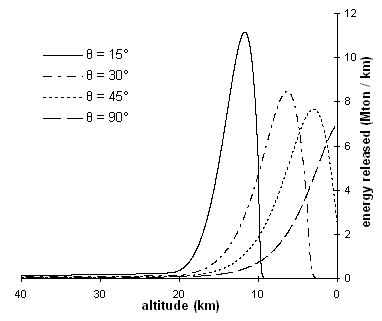Fig. 3: dEkin/dh vs. altitude for different initial &theta, stony impactors with v0 = 30 km/s.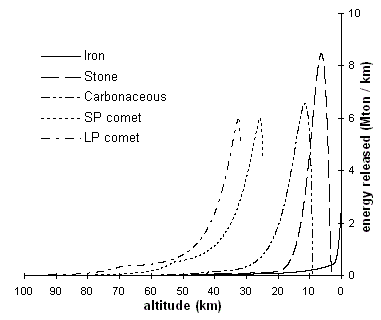Fig. 4: dEkin/dh vs. altitude for different initial compositions, Ekin0 = 60 MTon, θ0 = 30°.

The objects shown in figure 2 fragment shortly before the peak in dEkin/dh. After the fragmentation condition (Eq. 7) holds, the cross-sectional area A of a bolide begins to increase much more rapidly (according to Eq. 9), so that energy loss due to ablation (Eq. 3) and deceleration (Eq. 2), both proportional to A tends to increase much more rapidly as well. This can be interpreted as an explosion of the bolide, since it nearly depletes the object's initial kinetic energy, while the object deforms to a cross-sectional radius about 4 times larger than its initial radius at the height of the peak according to our data. Past this point it is no longer valid to assume that the entire remaining mass has a single shock front of hot air, since individual fragments might be far enough apart to develop their own shock fronts. Hills et. al uses a more involved procedure with three steps: pre-fragmentation dynamics, post-fragmentation dynamics as described above, but while there is still a single shock front, and then again pre-fragmentation dynamics for each piece of the bolide that now has its own wake, but achieves similar numeric results to Chyba et. al, whose model for deformation we have described in Eq. 7-9.

Figure 2 reveals that objects with higher initial velocities actually fragment and release most of their kinetic energies at higher altitudes than slower objects, which can reach the Earth's surface with a large fraction of their kinetic energy remaining. Figure 3 shows that steeper angles of entry, as measured from the horizon, result in deeper atmospheric entry prior to explosion. For the sets of input parameters shown here, variation in &theta(t) remained small (< 1%) until most (> 99%) of the bolide's kinetic energy was depleted or until impact with the Earth's surface (h = 0). Note that in Fig. 3, but not Fig. 2, each curve corresponds to the same initial kinetic energy of 60 Mton since Ekin is independent of &theta, so that lower peaks in dEkin/dh result in a broader altitude range for the explosion.

Fig. 4 is of particular interest for the discussion of the Tunguska and Lugo bolides, as it compares explosion altitudes for different material compositions: iron, stone, porous carbonaceous chondrite, and the less dense comets. Short period (SP) and long period (LP) comets do not differ in composition, but in their typical initial entry speeds, the latter twice as fast on average. These materials have varying heat of ablation Q, density, and yield strength S, as summarized in the table below.

Object Type Density (kg/m3) Heat of Ablation (J/kg) Yield strength (N/m2)
Iron 7900 8 x 106 108
Stone 3500 8 x 106 107
Carbonaceous 2200 5 x 106 106
Comet 1000 2.5 x 106 105

All plots in Fig. 4 correspond to the same initial kinetic energy of 60 Mton. Notice that for the SP and LP comets the plot terminates abruptly at about 30 km altitude. This is because the comets had their mass ablated to 0 at this altitude, so we terminated the simulation. We see that for the same initial kinetic energy, the more dense objects penetrate farther into the atmosphere before exploding, while iron objects with this energy are likely to impact the ground while retaining a large fraction of their initial kinetic energy (about 87% in this case).

## The Tunguska and Lugo Bolides

### Tunguska

On June 30, 1908 an explosion occurred over Tunguska, an area of Siberia. The primary pieces of evidence for this were eyewitness reports of a meteor, reports of a sound shockwave hundreds of km away, seismogram readings, and, after Leonid Kulik's 1930 expedition to the site below the explosion, the pattern of trees felled by the explosion in an area of 2150 km2 [Korobeinikov]. Because some trunks remained standing without any branches in an area well inside the flattened patch of forest, the object likely exploded prior to hitting the surface of the Earth and directly over the still-standing trunks. Estimation of the initial parameters of the Tunguska object has been difficult because eyewitness accounts, mostly used to determine incidence angle, were only collected decades after the fact, while the seismogram readings were of low accuracy and measured only the energy released by the shockwave of air impacting the ground near the epicenter of the explosion.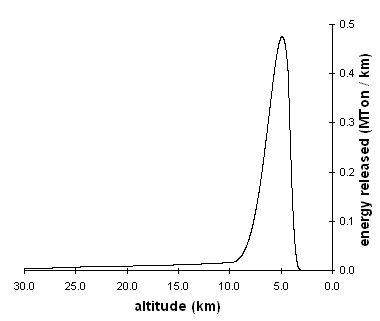Fig. 5: dEkin/dh vs. altitude for proposed Tunguska object.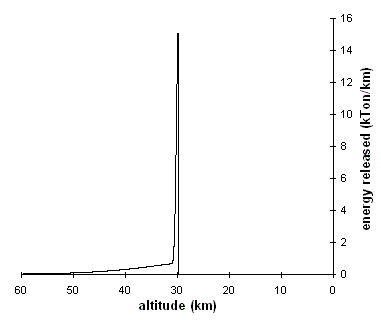Fig. 6: dEkin/dh vs. altitude for proposed Lugo object.

This initial shock front of air actually extinguished the forest fire ignited by the radiation released by the bolide, resulting in the charred, but preserved forest in the area of the explosion - each felled tree revealing the local direction of shock front propagation. Korobeinikov et. al simulated the tree fall pattern and found the initial &theta in the range 30°-45°, but do not compute the total kinetic energy dissipated in the event. The seismic records have been used to estimate this energy to about 15 Mton to within 25% certainty [Chyba], while the arrival time of the shock front in Irkutsk has been used by Ben-Menahem to estimate the height of the explosion to be about 8 km. Assuming a typical asteroid velocity of 15 km/s  and &theta = 30°, Fig. 5 shows the calculated release of energy vs. altitude for a proposed stony impactor.

The result is consistent with an explosion height of about 8 km and further supports the theory that the Tunguska object was a stony asteroid, since comets and carbonaceous meteors explode higher in the atmosphere, while iron objects are likely to impact the surface with much of their kinetic energy remaining (see Fig. 4 for a comparison at energies on the scale of tens of MTon).

### Lugo

Finally we apply this method to the Lugo bolide, which exploded over Italy in 1993. Much more is known about this event than Tunguska: the angle of incidence is reported at 8°-20° (we used 15°), initial speed 18±3 km/s, explostion height 30±3 km, and initial energy 14±2 kTon. If the object was carbonaceous in composition, we obtain the following curve for energy released vs. altitude for the Lugo bolide (Fig. 6). We see that the calculated explosion height does indeed match the observed height, supporting the conclusion, reached by a different analysis by Foschini, that the object was carbonaceous in composition.

© 2007 Dmitri Pavlichin. The author grants permission to copy, distribute and display this work in unaltered form, with attribution to the author, for noncommercial purposes only. All other rights, including commercial rights, are reserved to the author.

## References

 Q. R. Passey and H.J. Melosh, "Effects of Atmospheric Breakup on Crater Field Formation," Icarus 42, 211 (1980).

 J. G. Hills and M. P. Goda, "The Fragmentation of Small Asteroids in the Atmosphere," Astr. J. 105, 1114 (1993).

 C. F. Chyba, P. J. Thoams, and K. J. Zahnle, "The 1908 Tunguska explosion: atmospheric disruption of a stony asteroid," Nature 361, 40 (1993).

 V. A. Bronshten, Physics of Meteoric Phenomena, (Reidel, Holland 1983).

 V. P. Korobeinikov, P. I. Chushkin, and L. V. Shursalov, "Mathematical Model and Computation of the Tunguska Meteorite Explosion," Acta Astronautica 3, 615 (1976).

 L. Foschini, "On the Airburst of Large Meteoroids in the Earth's Surface," Astron. Astrophys. 337, L5 (1998).